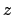Next: menu Up: Tcl Text Commands Previous: material   Contents   Index

## measure

The meaure command supplies several algorithms for analyzing molecular structures. In the following options, selection refers to an atom selection, as returned by the atomselect command described in section 8.3.2. The optional weight must be either none, an atom selection keyword such as mass, or a list of values, one for each atom in the selection, to be used as weights. If weight is missing or is none, then all weights are taken to be 1. When an atom selection keyword is used, the weights are taken from selection1.

• center selection [weight weight]: Returns the geometric center of atoms in selection using the given weight.

• fit selection1 selection2 [weight weight]: Returns a 4x4 transformation matrix which, when applied to the atoms in selection1, minimizes the weighted RMSD between selection1 and selection2. See section 11.4.2 for more on RMSD alignment.

• hbonds cutoff angle selection1 [selection2]: Find all hydrogen bonds in the given selection(s), using simple geometric criteria. Donor and acceptor must be within the cutoff distance, and the angle formed by the donor, hydrogen, and acceptor must be less than angle from 180 degrees. Only non-hydrogen atoms are considered in either selection. If both selection1 and selection2 are given, the selection1 is considered the donor and selection2 is considered the acceptor. If only one selection is given, all non-hydrogen atoms in the selection are considered as both donors and acceptors. The two selections must be from the same molecule. The function returns three lists; each element in each list corresponds to one hydrogen bond. The first list contains the indices of the donors, the second contains the indices of the acceptors, and the third contains the index of the hydrogen atom in the hydrogen bond.

Known Issue: The output of hbonds cannot be considered 100% accurate if the donor and acceptor selection share a common set of atoms.

• inverse matrix: Returns the inverse of the given 4x4 matrix.

• contacts cutoff selection1 [selection2]: Find all atoms in selection1 that are within cutoff of any atom in selection2 and not bonded to it. If selection2 is omitted, it is taken to be the same as selection1. selection2 and selection1 can either be from the same of from different molecules. Returns two lists of atom indices, the first containing the first index of each pair (taken from selection1) and the second containing the second index (taken from selection2). Note that the index is the global index of the atom with respect to its parent molecule, as opposed to the index within the given atom selection that contains it.

• minmax selection: Returns two vectors, the first containing the minimum,, andcoordinates of all atoms in selection, and the second containing the corresponding maxima.

• rmsd selection1 selection2 [weight weight]: Returns the root mean square distance between corresponding atoms in the two selections, weighted by the given weight. selection1 and selection2 must contain the same number of atoms (the selections may be from different molecules that have different numbers of atoms).

• rgyr selection [weight weight]: Returns the radius of gyration of atoms in selection using the given weight. The radius of gyration is computed as(8.1)

whereis the position of theth atom andis the weighted center as computer by measure center.

• sasa selection [-points varname] [-restrict restrictedsel] [-samples numsamples]: Returns the solvent-accessible surface area of atoms in the selection using the assigned radius for each atom, extending each radius by srad to find the points on a sphere that are exposed to solvent. If the restrictedsel selection is used, only solvent-accessible points near that selection will be considered. The restrict option can be used to prevent internal protein voids or pockets from affecting the surface area results. The points option can be used to see where the area contributions are coming from, and then the restrict flag can be used to eliminate any unwanted contributions after visualizing them. The varname parameter can be used to collect the points which are determined to be solvent-accessible.Next: menu Up: Tcl Text Commands Previous: material   Contents   Index
vmd@ks.uiuc.edu